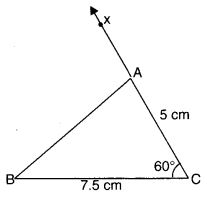NCERT Solutions for Class 7 Maths Chapter 10 Practical Geometry Ex 10.3 are part of NCERT Solutions for Class 7 Maths. Here we have given NCERT Solutions for Class 7 Maths Chapter 10 Practical Geometry Ex 10.3.

 Board CBSE Textbook NCERT Class Class 7 Subject Maths Chapter Chapter 10 Chapter Name Practical Geometry Exercise Ex 10.3 Number of Questions Solved 3 Category NCERT Solutions

## NCERT Solutions for Class 7 Maths Chapter 10 Practical Geometry Ex 10.3

Question 1.
Construct ADEF such that DE = 5 cm, DF 3 cm, and m ∠EDF = 90°.
Solution:
Steps of Construction:1. Draw a line segment DE = 5cm.
2. Draw ∠EDX = 90°.
3. With centre D and radius = 3 cm, draw an arc to intersect DX at F.
4. Join EF to obtain the required triangle DBF.

Question 2.
Construct an isosceles triangle in which the length of each of its equal sides is 6.5 cm and the angle between them is 110°.
Solution:
Steps of Construction

1. Draw a line segment QR of length 6.5 cm.
2. At Q, draw QX making 110° with QR, using a protractor.3. With Q as centre, draw an arc of a radius of 6.5 cm. It cuts QX at P.
4. Join PR. ∆ PQR is now obtained.

Question 3.
Construct ∆ ABC with BC = 7.5 cm, AC = 5 cm and m ∠C = 60°.
Solution:
Steps of Construction:1. Draw a line segment BC = 7.5 cm.
2. Draw ∠BCX = 60°.
3. With C as centre and radius = 5 cm, draw an arc intersecting CX at A.
4. Join AB to obtain the required ∆ABC.

We hope the NCERT Solutions for Class 7 Maths Chapter 10 Practical Geometry Ex 10.3 helps you. If you have any query regarding NCERT Solutions for Class 7 Maths Chapter 10 Practical Geometry EX 10.3, drop a comment below and we will get back to you at the earliest.